Theory and Modern Applications

# Application of Hilbert transform to fault detection in electric machines

## Abstract

In this study we investigate the possibility of broken bar fault detection in an induction machine rotor using spectral analysis of the stator currents. The numerical method, presented in this work, based on the Hilbert transform shows the possibility of improving the detection of faults in electrical machines.

The results obtained using this method are validated experimentally on a test bench with an induction motor of 5.5 kW.

## 1 Introduction

To detect the presence of faults in an induction machine rotor, diagnostic methods are classically based on frequency signals analysis of revealing signals . It is usual to use the modulus of the Fourier transform of the current absorbed by the machine to detect the presence of this type of failure . Indeed, a comparison of the amplitude of the fault signatory components with a reference threshold (threshold calculated when the machine is healthy) is used to detect the presence of an abnormality in the rotor electrical circuits.

In this part, special attention is paid to the contents of the phase spectrum of the stator current. This representation is generally used in image processing where the phase of the analyzed signal contains more relevant information than the module.

In this study, we show that it is possible to improve the diagnosis of the machine by exploiting the information given by the Hilbert transform applied to the amplitude spectrum of the stator current.

## 2 Hilbert transform

The Hilbert transform of a real-dimensional signal $y\left(t\right)$ can be calculated using the relation

$u\left(t\right)=-\frac{1}{\pi }{\int }_{-\mathrm{\infty }}^{+\mathrm{\infty }}\frac{y\left(\eta \right)}{\eta -t}\phantom{\rule{0.2em}{0ex}}d\eta =\frac{1}{\pi }{\int }_{-\mathrm{\infty }}^{+\mathrm{\infty }}\frac{y\left(\eta \right)}{t-\eta }\phantom{\rule{0.2em}{0ex}}d\eta .$
(1)

The inverse Hilbert transform is given by (1)

$y\left(t\right)=-\frac{1}{\pi }{\int }_{-\mathrm{\infty }}^{+\mathrm{\infty }}\frac{u\left(\eta \right)}{\eta -t}\phantom{\rule{0.2em}{0ex}}d\eta =\frac{1}{\pi }{\int }_{-\mathrm{\infty }}^{+\mathrm{\infty }}\frac{u\left(\eta \right)}{t-\eta }\phantom{\rule{0.2em}{0ex}}d\eta .$
(2)

Generally, the Hilbert transform is expressed using the convolution representation as follows: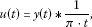(3)(4)

Contrary to the Fourier transform, which converts a signal from time domain into a signal in the frequency domain, the Hilbert transform does not change the domain of the variable. Indeed, the Hilbert transform of a t-dependent signal is also a function of the same variable t. The Fourier transform of the core of the Hilbert transform, i.e., $\ominus t=\frac{1}{\pi \cdot t}$ (3) and (4) is

$\ominus t=\frac{1}{\pi \cdot t}\stackrel{\mathrm{TF}}{⟶}-j\cdot sgn\left(\omega \right),$
(5)

where the sign function, denoted sgn, is given by the following values:

(6)

The multiplication of this kernel by the convolution theorem from Fourier analysis, gives the spectrum of the Hilbert transform:

$u\left(t\right)\stackrel{\mathrm{FT}}{⟶}V\left(\omega \right)=j\cdot sgn\left(\omega \right)\cdot Y\left(\omega \right).$
(7)

This relation allows the calculation of the Hilbert transform from the inverse Fourier transform of the spectrum given by the above equation

$V\left(\omega \right)=j\cdot sgn\left(\omega \right)\cdot Y\left(\omega \right)\stackrel{{\mathrm{FT}}^{-1}}{⟶}u\left(t\right)$
(8)

(${\mathrm{FT}}^{-1}$: inverse Fourier transform).

The calculation of the Fourier transform can be done using the algorithms DFT (Discrete Fourier Transform) or FFT (Fast Fourier Transform). In general, a real signal $y\left(t\right)$ can be decomposed into a sum of two terms

$y\left(t\right)={y}_{p}\left(t\right)+{y}_{i}\left(t\right),$
(9)

where the even term is

${y}_{p}\left(t\right)=\frac{y\left(t\right)+y\left(-t\right)}{2}$
(10)

and the odd term is

${y}_{p}\left(t\right)=\frac{y\left(t\right)-y\left(-t\right)}{2}.$
(11)

The Fourier transform of $y\left(t\right)$ is a complex function that can be put in the form

$Y\left(\omega \right)=\mathrm{\Re }\left(Y\left(\omega \right)\right)+j\cdot \mathrm{\Im }\left(Y\left(\omega \right)\right)={Y}_{\mathrm{Re}}\left(\omega \right)+j\cdot {Y}_{\mathrm{Im}}\left(\omega \right).$
(12)

Multiplying the Fourier transform $Y\left(\omega \right)$ by the operator $-j\cdot sgn\left(\omega \right)$ changes the real part into an imaginary part and vice versa (7). The spectrum of the Hilbert transform, meanwhile, can get the form

$V\left(\omega \right)=\mathrm{\Re }\left(V\left(\omega \right)\right)+j\cdot \mathrm{\Im }\left(V\left(\omega \right)\right)={V}_{\mathrm{Re}}\left(\omega \right)+j\cdot {V}_{\mathrm{Im}}\left(\omega \right).$
(13)

Or, based on previous demonstrations, we have

${V}_{\mathrm{Re}}\left(\omega \right)=-j\cdot sgn\left(\omega \right)\left[j\cdot {Y}_{\mathrm{Im}}\left(\omega \right)\right]=sgn\left(\omega \right)\cdot {Y}_{\mathrm{Im}}\left(\omega \right)$
(14)

and

${V}_{\mathrm{Im}}\left(\omega \right)=-j\cdot sgn\left(\omega \right)\cdot {Y}_{\mathrm{Re}}\left(\omega \right).$
(15)

Therefore, we can notice that the Hilbert transform changes all the even terms into odd terms and all the odd terms into even terms. We can then give the Hilbert transform of cosine and sine harmonic functions as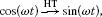(16)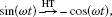(17)(18)

From these equations, we can notice that the Hilbert transform changes all cosine terms into sine terms and all sine terms into negative cosine terms. The Hilbert transform in the time domain corresponds to a phase shift of value $\frac{\pi }{2}$ of all the terms of the Fourier transform.

## 3 Hilbert transform in the theory of modulation

In addition to the $\frac{\pi }{2}$ phase shift that it introduces between the real and imaginary parts, the Hilbert transform can be used in the theory of modulation to determine the amplitude modulation, phase modulation and frequency modulation of a time-dependent signal $y\left(t\right)$.

The Hilbert transform of a signal $y\left(t\right)$ can be written as

$y\left(t\right)\stackrel{\mathrm{HT}}{⟶}\stackrel{˜}{y}\left(t\right)={\stackrel{˜}{y}}_{\mathrm{Re}}\left(t\right)+j\cdot {\stackrel{˜}{y}}_{\mathrm{Im}}\left(t\right),$
(19)

where ${\stackrel{˜}{y}}_{\mathrm{Im}}\left(t\right)$ represents the Hilbert transform of the signal ${\stackrel{˜}{y}}_{\mathrm{Re}}\left(t\right)$. The signal $\stackrel{˜}{y}\left(t\right)$, meanwhile, is commonly called an analytic signal.

The amplitude $A\left(t\right)$ of the time signal $y\left(t\right)$ is calculated using the following relation:

$A\left(t\right)=\sqrt{{\stackrel{˜}{y}}_{\mathrm{Re}}^{2}\left(t\right)+{\stackrel{˜}{y}}_{\mathrm{Im}}^{2}\left(t\right)}.$
(20)

Its phase $\phi \left(t\right)$ is calculated using the relation

$\phi \left(t\right)=arctan\frac{{\stackrel{˜}{y}}_{\mathrm{Im}}\left(t\right)}{{\stackrel{˜}{y}}_{\mathrm{Re}}\left(t\right)}.$
(21)

From this phase, we can determine the expression for calculating the frequency

$F\left(t\right)={F}_{0}+\frac{1}{2\pi }\frac{d\phi \left(t\right)}{dt}.$
(22)

If we take as an example a function $y\left(t\right)$ modulated in amplitude and in phase with the expression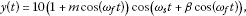(23)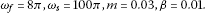(24)

Calculating the Hilbert transform of this signal gives an analytical complex signal $\stackrel{˜}{y}\left(t\right)$ with real part ${\stackrel{˜}{y}}_{\mathrm{Re}}\left(t\right)$ and imaginary part ${\stackrel{˜}{y}}_{\mathrm{Im}}\left(t\right)$ (19).

## 4 Experimental results

The experimental bench consists of a squirrel cage induction motor of 5.5 kW (Figure 1). The engine is Leroy Somer LS 132S, IP 55, class F, ${T}^{\circ }\text{C}={40}^{\circ }\text{C}$. The nominal voltage between phases 400 V, supply frequency 50 Hz, speed rate 1440 r/min, the number of slots in the rotor $\mathit{Nr}=28$. The number of slots in the stator $\mathit{Ns}=48$. The stator windings are star-coupled . The motor is loaded with a powder brake. Its maximum torque (100 Nm) is reached at the nominal speed.

### 4.1 Rotor fault influence on the stator current spectrum phase

The spectrum modulus and phase of the stator current of a rotor cage with four broken bars (4b-C100) (Figure 2) (connection to a 3-phase supply) are shown in Figures 3 and 4. It is clear that the frequency components $\left(1±2kg\right)fs$ are present in the amplitude spectrum of the stator current as it is shown in Figure 3.

To be sure that the phase jumps of frequencies $\left(1±kg\right)fs$ present in this spectrum are due to the presence of a damaged rotor bar, we compared with the spectrum of the stator current when the induction machine operates with a healthy rotor. This analysis helps to reinforce the fact that the appearance of a broken bar in the machine rotor leads to picks in the spectrum at frequencies $\left(1±2kg\right)fs$ .

We have shown that the analysis of the stator current spectrum tells us about the rotor state of the induction machine.

We notice that the jumps of the spectrum present at frequencies $\left(1±2kg\right)fs$ were clearly due to the presence of one or more damaged rotor bars. Therefore, based on this information, it is possible to establish a diagnosis of squirrel cage by analyzing the spectrum of particular picks.

To undertake a rotor fault diagnosis without need for comparison with a reference (reference obtained from a healthy functioning) , the final decision, that is, ‘Is the rotor healthy or not?’, must be made exclusively from the analyzed signal. This will allow us to apply the method to low or high power machines. We know that all induction machines have a slight asymmetry of construction that induces, in the stator current spectrum, a frequency component $\left(1-2g\right)fs$. Sometimes, the oscillation speed created by this component is large enough to make an additional component of frequency $\left(1+2g\right)fs$ appear in the same frequency spectrum. However, induction motors manufacturers ensure that the machines present asymmetry as small as possible because it could be the main cause of faults . For instance, a static eccentricity causes a homopolar current enclosed in the bearings reduce their lifetime significantly . It is in this light that the diagnostic method will be developed. We study the stator current spectrum and especially the frequency jump at $\left(1+2g\right)fs$. Normally, this jump is very low or even zero for a healthy induction machine, and this is true whatever the charge is.

### 4.2 Hilbert transform for rotor faults diagnosis

This section develops the diagnosis method based on the calculation of the phase of the analytical signal obtained by a Hilbert transform of the spectrum amplitude of the current absorbed by the induction machine. In other words, instead of working directly on the stator current (time signal), we suggest working with the module of its Fourier transform. As we previously mentioned, the Hilbert transform of a signal returns a representation of this signal in the same domain. Thus, if we apply the Hilbert transform of the modulus of the Fourier transform of the stator current, the resulting signal will therefore be expressed in the frequency domain.

This approach uses the Hilbert transform calculated from the spectrum module of the stator current, its phase has no importance here. Figure 4 represents the analytic signal phase obtained by calculating the Hilbert transform of the spectrum module of the stator current, when the machine operates with a healthy rotor Figure 4(a) and a failing rotor Figure 4(b). These figures reveal the presence of ‘phase jumps’ at fault frequencies $\left(1±2kg\right)fs$. Moreover, we can notice that the appearance of the rotor fault increases the amplitude of the jumps present at phase $\phi \mathrm{HT}\left(f\right)$.

We can notice the presence of a rapid change in the phase at 50 Hz. As the phase of the FT of the current, having a clear phase change at 50 Hz, allows the evaluation of the amplitude of the phase jump at $\left(1-2g\right)fs$ more easily than the amplitude of component of the same frequency present in the stator current spectrum module Figure 4(b).

For our machine, there is no problem in the detection of this frequency, either in the spectrum amplitude or in the phase $\mathrm{HT}\left(f\right)$, but in the case of high power motors, this detection may be difficult due to the low slip value (about 1%) because of the dominance of the fundamental harmonic frequency 50 Hz.

The difference between the phase of the Fourier transform and the phase of the analytic signal lies in the fact that the latter is calculated from the spectrum amplitude of the stator current. This means that, as soon as the frequency component $\left(1-2g\right)fs$ appears in the spectrum module, it will also appear in the phase $\phi \mathrm{HT}\left(f\right)$. Even the component created by the rotor fault has a relatively low amplitude in the module of the stator current frequency spectrum, it appears in the phase of the analytic signal $\phi \mathrm{HT}\left(f\right)$ because the modulus of the spectrum contains this information. Moreover, it should be noted that the amplitude of the phase jumps located at frequencies $\left(1±2kg\right)fs$ of the phase $\phi \mathrm{HT}\left(f\right)$ is directly related to the amplitude of the components located at the same frequencies in the modulus of the spectrum of the stator current.

## 5 Conclusion

In this work, we present a method based on the spectrum analysis of the stator current in the induction motor for detecting the presence of faults in the machine rotor. The use of the Hilbert transform of the spectrum amplitude of the stator current is used to detect the presence of any fault in the rotor. The experimental results conducted on a test bench show the effectiveness of this method.

## References

1. Didier, G: Modélisation et diagnostic de la machine asynchrone en présence de défaillances. Thèse de doctorat, Université Henri Poincaré, Nancy-I, France (2004)

2. Nus, P: Etude et implantation de méthodes d’estimation spectrale paramétrique et non-paramétriques Application à l’analyse vibratoire de machines tournantes. Thèse de doctorat, Université de Nancy-I (1993)

3. van Schoneveld C, Frijling J: Spectral analysis: on the usefulness of linear tapering for leakage suppression. IEEE Trans. Acoust. Speech Signal Process. 1981, 29(2):323-329. 10.1109/TASSP.1981.1163524

4. Capdessus, C: Aide au diagnostic des machines tournantes par traitement du signal. Thèse de doctorat, Institut National Polytechnique de Grenoble (1992)

5. Medoued A, Lebaroud A, Boukadoum A: On-line monitoring and diagnosis of broken rotor bars in induction motor. 6th International Conference on Electrical and Electronics Engineering ELECO ’09, Bursa, Turkey, 5-8 November 2009 (2009).

6. Milimonfared J, Kelk HM, Nandi S, Toliyat HA: A novel approach for broken-rotor-bar detection in cage induction motors. IEEE Trans. Ind. Appl. 1999, 35(5):1000-1006. 10.1109/28.793359

7. Didier G, Razik H, Rezzoug A: On the modelling of induction motor including the first space harmonics for diagnosis purposes. International Conference on Electrical Machines,August 2002 (2002).

8. Nandi S, Toliyat HA: Detection of rotor slot and other eccentricity related harmonics in a three phase induction motor with different rotor cages. IEEE Trans. Energy Convers. 2001, 16(3):253-260. 10.1109/60.937205

## Author information

Authors

### Corresponding author

Correspondence to A Medoued.

### Competing interests

The authors declare that they have no competing interests.

### Authors’ contributions

This work was performed in collaboration of all authors. AL collected experimental measurements, supervised the work, participated in the analysis of results and finalized the manuscript. AM carried out calculations, analyzed the calculated data, and drafted the manuscript. DS helped analyze the results, revised and corrected the English version of the manuscript. All authors read and approved the final manuscript.

## Authors’ original submitted files for images

Below are the links to the authors’ original submitted files for images.

## Rights and permissions

Reprints and Permissions

Medoued, A., Lebaroud, A. & Sayad, D. Application of Hilbert transform to fault detection in electric machines. Adv Differ Equ 2013, 2 (2013). https://doi.org/10.1186/1687-1847-2013-2